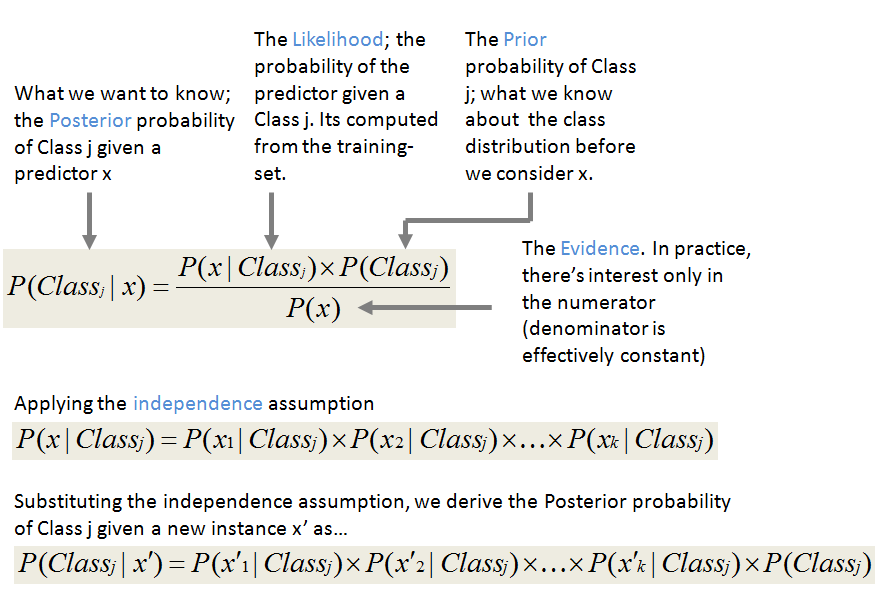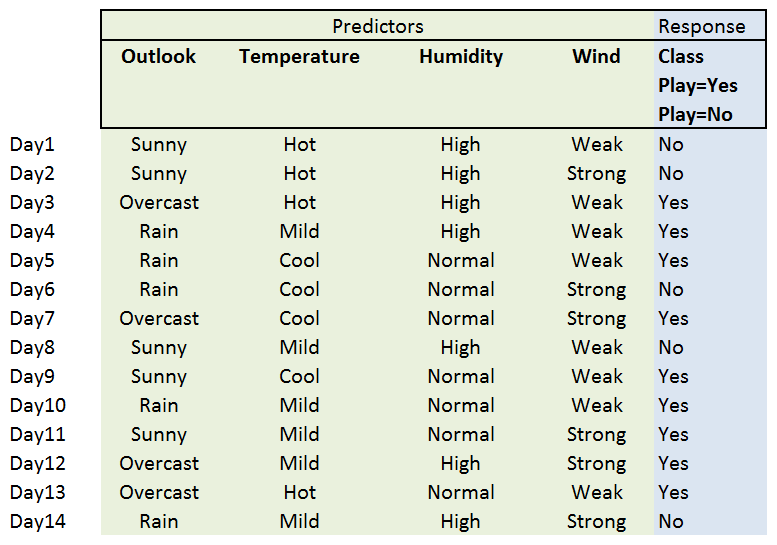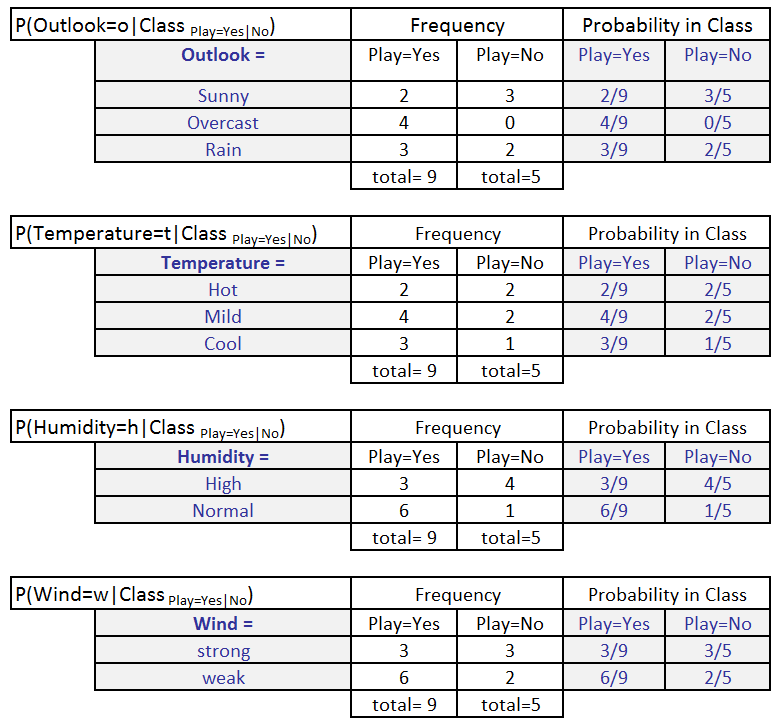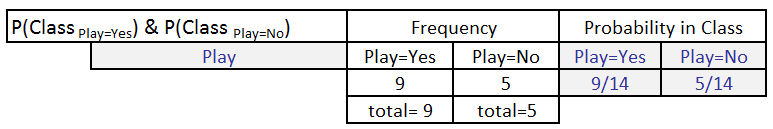# 09.12.13

## Not-so-Naive Classification with the Naive Bayes Classifier

Posted in Bayes Reasoning at 8:59 pm by Auro Tripathy

A common (and successful) learning method is the Naive Bayes classifier. When supplied with a moderate-to-large training set to learn from, the Naive Bayes Classifier does a good job of filtering out less relevant attributes and make good classification decisions. In this article, I introduce the basics of a  Naive Bayes classifier, provide an often-cited example, and provide working R code.

##### Introduction to Naive Bayes Classifiers

The Naive Bayes classifier is based on Bayes’ theorem with the independence assumptions between features.

####The Bayes’ rule (above) plays a central role in the probabilistic reasoning since it helps us ‘invert’ probabilistic relationships between P(Class | x ) and P(x | Class).

##### So what’s naive about Naive Bayes?

It naively assumes that the attributes of any instance of the training-set are conditionally independent of each other (in our example below, the cool temperatures are completely independent of the sunny outlook). We represent this independence as:

P(x1, x…, x| Classj) = ∏i P(xi, | Classj), or

P(x1, x…, x| Classj) = P(x| Classj) × P(x| Classj) ×…× P(x| Classj)

In plain English, if each feature (predictor) x is independent of every other  feature, then the probability a data-point  (x1, x…, xk) is in Classj is simply the product of  all the individual probabilities of feature xi in Classj.

#### Example

Let’s build a classifier that predicts whether I should play tennis given the forecast. It takes four attributes to describe the forecast; namely, the outlook, the temperature, the humidity, and the presence or absence of wind. Furthermore the values of the four attributes are qualitative (also known as categorical). They take on the values shown below.

Outlook ∈ [Sunny, Overcast, Rainy]
Temperature ∈ [Hot, Mild, Cool]
Humidity ∈ [High, Normal]
Windy ∈ [Weak, Strong]

The class label is the variable, Play and takes the values yes or no.

Play∈ [Yes, No]

We read-in training data below that has been collected over 14 days.##### The Learning Phase

In the learning phase, we compute the table of likelihoods (probabilities) from the training data. They are:

P(Outlook=o|ClassPlay=b), where o ∈ [Sunny, Overcast, Rainy] and b ∈ [yes, no]

P(Temperature=t|ClassPlay=b), where t ∈ [Hot, Mild, Cool] and b ∈ [yes, no],

P(Humidity=h|ClassPlay=b), where h∈ [High, Norma] and b ∈ [yes, no],

P(Wind=w|ClassPlay=b), where w ∈ [Weak, Strong] and b ∈ [yes, no].We also calculate P(ClassPlay=Yes) and P(ClassPlay=No).##### Classification Phase

Let’s say, we get a new instance of the weather condition, x’=(Outlook=Sunny, Temperature=Cool, Humidity=High, Wind=Strong) that will have to be classified (i.e., are we going to play tennis under the conditions specified by x’).

With the MAP rule, we compute the posterior probabilities. This is easily done by  looking up the tables we built in the learning phase.

P(ClassPlay=Yes|x’) = [P(Sunny|ClassPlay=Yes) × P(Cool|ClassPlay=Yes) ×

P(High|ClassPlay=Yes) × P(Strong|ClassPlay=Yes)] ×

P(ClassPlay=Yes)

= 2/9 × 3/9 × 3/9 × 3/9 × 9/14 = 0.0053

P(ClassPlay=No|x’) =  [P(Sunny|ClassPlay=No) ×P(Cool|ClassPlay=No) ×

P(High|ClassPlay=No) × P(Strong|ClassPlay=No)] ×

P(ClassPlay=No)

= 3/5 × 1/5 × 4/5 × 3/5 × 5/14 = 0.0205

Since P(ClassPlay=Yes|x’) less than P(ClassPlay=No|x’), we classify the new instance x’ to be “No”.

#### The R Code

The R code works with the example dataset above and shows you a programmatic way to invoke the Naive Bayes classifier in R.

```rm(list=ls())
library(e1071) #naive Bayes classifier library
classifier<-naiveBayes(tennis.anyone[,1:4], tennis.anyone[,5])
table(predict(classifier, tennis.anyone[,-5]), tennis.anyone[,5], dnn=list('predicted','actual'))
classifier\$tables
#new data #15
tennis.anyone[15,-5] <- as.factor(c(Outlook = "Sunny", Temperature = "Cool", Humidity = "High", Wind = "Strong"))

print(tennis.anyone[15,-5] )

result <- predict(classifier, tennis.anyone[15,-5] )
print(result)```

Created by Pretty R at inside-R.org

#### Things t0 watch-out for – data underflow during multiplications

Calculating the product below may cause underflows.

P(x| Classj) × P(x| Classj) ×…× P(x| Classj) × P(Classj).

You can easily side-step the issue by moving the computation to the logarithmic domain.
log(P(x| Classj) × P(x| Classj) ×…× P(x| Classj) × P(Classj)) =
log(P(x| Classj)) + log(P(x| Classj)) +…+ log(P(x| Classj)) + log(P(Classj))

#### References

Bayesian Reasoning and Machine Learning, by David Barber

http://www.csc.kth.se/utbildning/kth/kurser/DD2431/mi07/07_lecture07_6.pdf

http://www.cs.nyu.edu/faculty/davise/ai/bayesText.html